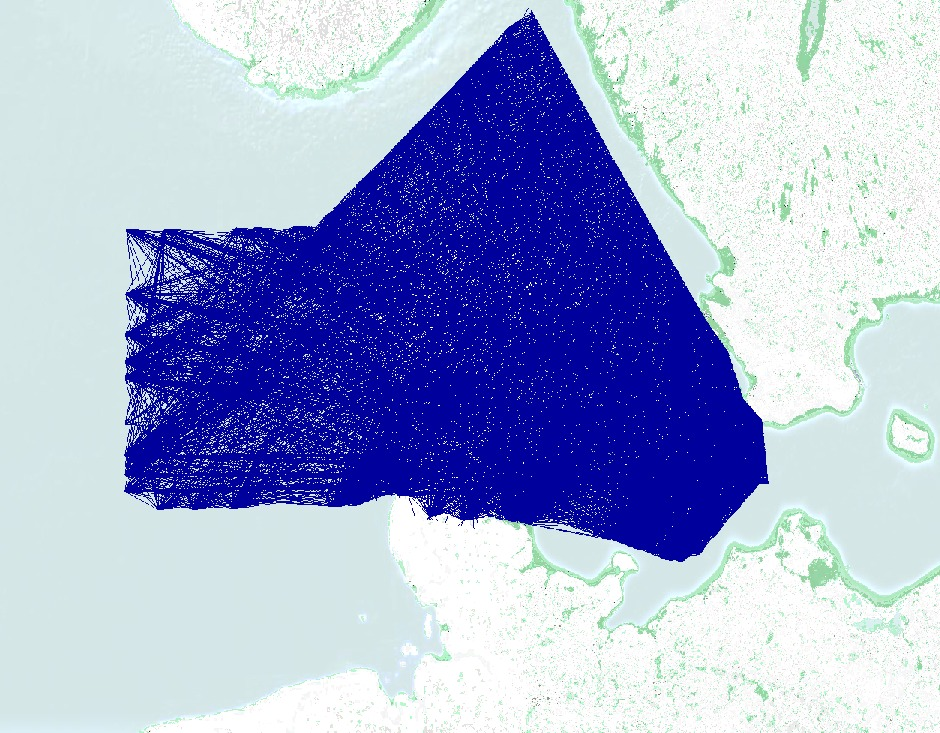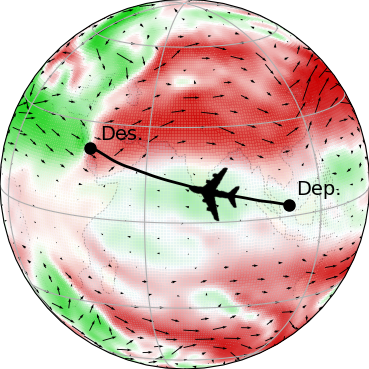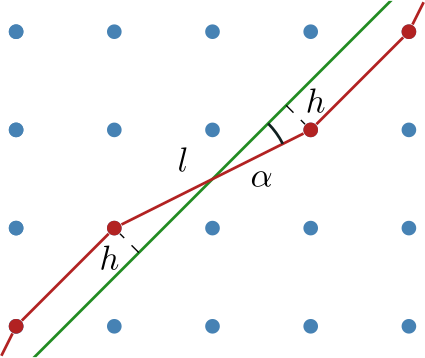The current system of routing flights on predefined airway networks does not harvest the benefits of exploiting tailwinds in an optimal way. Dropping the restriction to airways allows saving fuel and reducing CO2emission – an important driver in view of growing air traffic. Established graph-based routing algorithms face considerable computational challenges in this setting. At ZIB, we develop and analyze hybrid approaches combining discrete optimization algorithms with optimal control methods in order to compute globally optimal free flight trajectories to high accuracy in an efficient way.

Established flight planning involves shortest path selection in a pre-defined airway network, where effective edge lengths depend also on the wind speed at the time the plane passes that edge. In order to reduce fuel costs and air pollution, free flight zones are increasingly discussed. Removing the restriction of following the pre-defined airways allows taking better routes and exploiting advantageous wind situations more effectively. Achieving this flexibility with established graph-based optimization methods requires a massive refinement of the graph leading to significantly increased computing times.

How much can one gain? Practical experience, recent studies, and our own numerical examples indicate potential savings in fuel and CO2 emission of about 2%, up to 10% in extreme cases. As an example, consider the figure to the right. The optimal free flight route (in green) is not only faster, but also smoother in operation.Free Flight approximation of the Danish airspace by using a denser graph.

To harvest these benefits, flight planning tools are needed that can find the quickest, most fuel efficient, etc. free flight route with respect to the weather conditions, and with respect to a number of other side constraints such as traffic rules, in reasonable time. For some time now, gradually expanding free flight opportunities have been successfully approximated by graph densification, see Figure to the left. It is intuitively clear, however, that this approach does not scale well. As Dijkstra-based algorithms solve time-dependent shortest path problems usually with a time-complexity of O(|E| + |V| log |V|), at some point, the problem becomes intractable. All the more so when several Free Flight airspaces are merged.

Continous methods of optimal control, which have been studied for a century, suggest themselves as an algorithmic alternative. Instead of covering the airspace by a graph, they only discretize the trajectory itself, exploiting necessary optimality conditions to move the waypoints continuously in space, see Figure to the right. Consequently these methods scale much better than global graph-based approaches, i.e. the run time complexity in terms of the desired accuracy is much lower.

The downside of that approach is, that these methods only converge to some nearby local optimum, if at all, and thus cannot guarantee global optimality. Thus, in itself, optimal control approaches are insufficient for free-flight planning.
Can discrete and continuous methods be merged into an approach that combines their strengths and eliminates their weaknesses, i.e., a method that provides, at the same time, global optimality and rapid convergence to an optimal free flight trajectory, at any desired accuracy? Exactly this is the aim of a new method that is currently developed at ZIB: We recently proposed a novel hybrid algorithm under the name DisCOptER (Discrete-continuous optimization for enhanced resolution) [BorndörferDaneckerWeiser2021].

### DisCOptER – Algorithm

While discrete, graph-based approaches efficiently approximate global optima at low to medium accuracy, continuous optimal control methods excel at fast local convergence to high accuracy. The hybrid DisCOptER method exploits these properties in a two-stage approach. In a nutshell, it starts an optimal control algorithm from a suitable path provided by a discrete dynamic programming method.

First, an artificial, locally connected graph is created that contains origin and destination vertices (cf. Video to the right, blue dots. Assume that each dot is connected to every other one.), and a shortest path on this graph is calculated (red path). Finally, this shortest path is used as an initial guess for the following refinement stage of the algorithm. Here, an optimal control approach involving some variant of Newton’s method is used to calculate a highly accurate solution (green trajectory). Provided that the first guess is within the radius of convergence of the actual continuous optimum (green area), Newton’s method converges extremely fast.The optimal trajectory from Brunei to Jeddah exploits tailwind (green) and avoids headwind regions (red) as far as possible.

For the second stage to converge reliably to the global optimum in the vicinity of the path provided by the first stage, this path must be sufficiently close to the global optimum, more precisely, within the domain of convergence of Newton’s method. This can be ensured by making the first-stage graph sufficiently dense. The required graph resolution depends only on external factors like the wind conditions, but not on the desired accuracy, which only affects the trajectory discretization in the second stage.

It turns out, that in practically relevant examples the required graph density is rather low (again, see the video above). Consequently, the discrete shortest path can be found quickly, the problematic asymptotic runtime behavior of the discrete graph search is circumvented and the overall time complexity is governed by the asymptotically efficient optimal control stage (Time complexity of the purely discrete approach: O(l^-6) vs. the hybrid algorithm O(l^-1), where 1/l is a measure for the solution accuracy). But can we decide within the algorithm, how dense the graph must be in a concrete case?

For free flight trajectory planning, the graph used either stand-alone for path planning or as the first stage in a hybrid algorithm needs to be sufficiently fine for the discrete optimal path to be accurate enough or to lie within the convergence domain of Newton’s method, respectively. To ensure this, a priori error estimates for optimal flight paths in locally densely connected graphs have been derived [BorndörferDaneckerWeiser2021-b]. (h,l)-dense graphs are those where the h-balls around vertices cover the plane, and all vertices with a distance of at most l+2h are connected by an edge, see the Figure to the right.

Following the standard paradigm of a priori error estimates for finite element discretizations, the distance of an optimal continuous path to its interpolate in the graph is bounded, in terms of the flight duration, by bounding the second derivative of the objective with respect to path deviations.

The structure of (h,l)-dense graphs guarantees the existence of a reasonable interpolant. Since the optimal discrete path in the graph is not worse than the considered interpolant, this provides an error bound for the discrete solution in terms of vertex density h, local connectivity length l, and magnitudes of wind speed and its spatial derivatives.Angular (top) and distance error (bottom) between a discrete (red) and a continuous route (green).

The excess in flight duration is due to spatial displacement of the trajectory on one hand, and zig-zagging due to limited angular resolution on the other hand. The latter is bounded by l/h, whereas the former is controlled by h, see the Figures to the left. Based on this distinction, the error estimate allows to select a theoretically optimal relation of vertex density h and connectivity radius l, which turns out to be h = O(l^2). The resulting error bound guarantees a convergence of optimal discrete paths towards the continuous optimum in terms of flight duration at a rate of O(l^2).

As usual, the a priori error bounds are reliable but far from sharp. Nevertheless, they capture the numerically observed convergence order accurately. This provides the basis on which efficient a posteriori error estimators can be designed, which can actually guide the graph refinement to the desired accuracy and allows implementing efficient and reliable hybrid algorithms.

DisCOptER is a first example of a new type of discrete-continuous algorithms that combine global optimality with fast local convergence. The underlying principle is not limited to shortest path problems, but general enough to be applicable to many other problems of similar nature.

Video: